# Lesson 40: How to enter and format Fractions in Excel

This article will show you how to enter a fraction in Excel and how to change the fraction format.

 Join the channel Telegram belong to AnonyViet 👉 Link 👈## How to enter and format fractions in Excel

first. To enter the mixed number 1 4/7 (one and four sevenths), type 1 4/7. To enter the fraction 4/7 (four sevenths) in cell A1, select cell A1 and enter 0 4/7.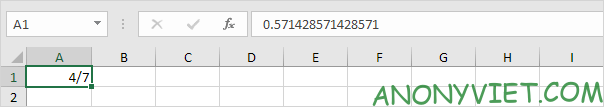Now that you can enter an exact fraction, Excel has applied the Fraction format to cell A1, and you can edit the fraction by simply typing 5/7 (no zero). You can find the decimal value of the fraction in the formula bar.

2. See Fraction format. Select cell A1, right-click, and then click Format Cells.

Excel has applied Up to one digit (up to 1 digit).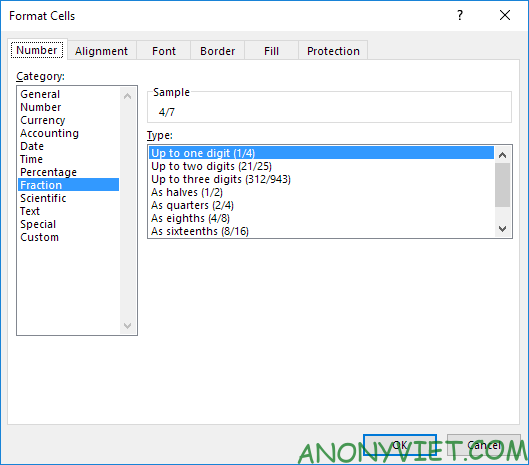Note: To enter a fraction like 4/11 in cell A1, change the type to Up to two digits. To enter a fraction like 1/148 in cell A1, change the type to Up to three digits.

3a. Excel rounds your fraction if it can’t display the right fraction. For example, if you enter 4/11 in cell A1 (with Up to one digit), Excel displays 1/3. The formula bar always shows the exact decimal value.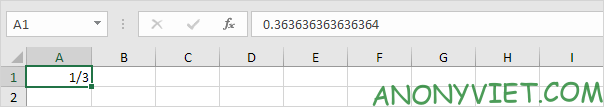3b. Change the format style to Up to two digits.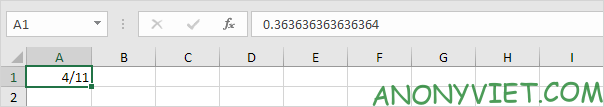Note: of course, if you enter 0 4/11 in a new cell, Excel will automatically apply the Up to two digits format and display 4/11 exactly.

4. Excel will always reduce fractions to the smallest denominator. If you enter the fraction 2/8, Excel converts the fraction to 1/4. However, you can use the As eights (4/8) format to correct the denominator to 8.

See more:  How to use PivotTable in Excel to make statistics and reports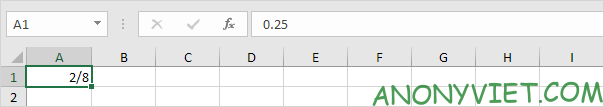5. Sometimes, you can’t find the right denominator for your fraction. For example, you want to display 40/50, but Excel converts 40/50 to 4/5. However, the As fiftieths (25/50) format is not available. You can create a format (# ?? / 50) to fix this.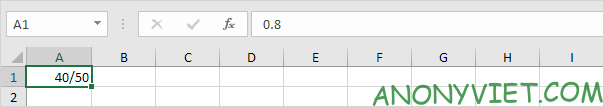Note: change 50 to 60 to make the format As sixtieths (30/60)…

In addition, you can also see many other excel articles here.

The article won: 1/5 – (999 votes)

Source: Lesson 40: How to enter and format Fractions in Excel
– TechtipsnReview